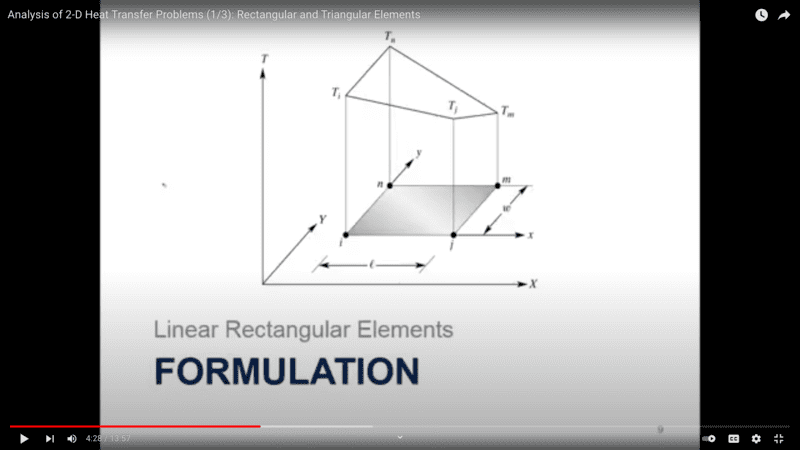# Solving 2D Heat Equation w/ FEM & Galerkin Method

• I
• bob012345
In summary, you are solving the Laplace equation in a rectangular region with known boundary conditions. You find a solution as a sum of solutions of the two problems given, and you have conceptual issues with the second problem around the 2D element.f

#### bob012345

Gold Member
TL;DR Summary
Solving a 2D temperature problem but having an issue with the proper setup of the Galerkin method. All my terms are zero.
I want to solve the 2D heat equation

$$\frac{∂^2 {T}}{ ∂x^2} + \frac{∂^2 {T}}{ ∂y^2} = 0$$

The only boundary conditions is I will specify the edge temperatures but there are no heat sources.

So I create an average temperature function ##\tilde{T}## and weighting functions ##S_i## over a rectangular element with four nodes with i=1,2,3,4.Using numbers instead of letters for clarity I wish to solve first for the weighting functions assuming a form for my average temperature function;
$$\tilde{T} = b_1 + b_2x +b_3y +b_4xy$$

I solve for the coefficients to get my weighting functions and my average temperature function;

$$S_1 = (1 - \frac{x}{l}) (1 - \frac{y}{w})$$
$$S_2 = \frac{x}{l}(1 - \frac{y}{w})$$
$$S_3 = \frac{xy}{lw}$$
$$S_4 = \frac{y}{w}(1 - \frac{x}{l})$$

Then;
$$\tilde{T} = T^e = S_1T_1 + S_2T_2 + S_3T_3 + S_4T_4$$

Following the Galerkin method I minimize the Residual

$$\iint_A S_i R \,dA = \iint_A S_i (\frac{∂^2 \tilde{T}}{ ∂x^2} + \frac{∂^2 \tilde{T}}{ ∂y^2} )\,dA = 0$$

Now I use integration by parts to eliminate the second order derivative. This is just for the x derivative part of one of the four equations;

$$\iint_A S_i \frac{∂^2 \tilde{T}}{ ∂x^2}\,dA = \int_Y \Big[ S_i \frac{∂ \tilde{T}}{ ∂x} \Big |_{x_1}^{x_2} - \int_X \frac{∂S_i}{∂x} \frac{∂ \tilde{T}}{ ∂x} \,dX \Big] dY$$ where the index goes to 4.

My problem is when I integrate this to get my node equations all the terms go to zero. I mean the two integrals exactly cancel for each node variable. My question is this, did I set up this correctly with the integration by parts over a double integral? I used the integration of parts for the x integral then when manipulating the x derivative terms. Thanks.

EDIT: I think I found the issue. It is how to evaluate the first term after integration by parts. I was substituting the full expression for ##\frac{∂ \tilde{T}}{ ∂x}## back in when it should be evaluated as the slope at the limits of integration. I found it by revisiting my 1D reference problem. Thanks.

Last edited:
•Delta2
Summary: Solving a 2D temperature problem but having an issue with the proper setup of the Galerkin method. All my terms are zero.

I want to solve the 2D heat equation

∂2T∂x2+∂2T∂y2=0
it is not the heat equation it is the Laplace

•berkeman and bob012345
it is not the heat equation it is the Laplace
There are no heat sources. Only boundary edge temperature constrains.

I understand. Nevertheless it is the Laplace equation.

•bob012345
I understand. Nevertheless it is the Laplace equation.
I agree but can you tell me how I should include the boundary conditions? I am a bit fuzzy on that. Should there be some form of source term? Thanks.

To solve the Laplace equation ##\Delta u=0## in a rectangular region $$0\le x\le a,\quad 0\le y\le b$$ with known boundary conditions
$$u(x,0)=\alpha(x),\quad u(0,y)=\beta(y),\quad u(a,y)=\gamma(y),\quad u(x,b)=\psi(x)$$
you can find a solution as a sum of solutions of the following two problems
$$\Delta u_1=0,\quad u_1(x,0)=0=u_1(x,b)=0,\quad u_1(0,y)=\beta(y),\quad u_1(a,y)=\gamma(y)$$
and
$$\Delta u_2=0,\quad u_2(x,0)=\alpha(x),\quad u_2(x,b)=\psi(x),\quad u_2(0,y)=0= u_2(a,y)$$
Further
$$u_1=\sum_{k=0}^\infty v_k(x)\sin(\pi k y/b),\quad u_2=\sum_{k=0}^\infty w_k(y)\sin(\pi k x/a)$$

Last edited:
•bob012345
oh, I have just noticed the word "FEM" that is not my field sorry

•bob012345
I resolved how to do the calculations for this part;

$$\int_X \frac{∂S_i}{∂x} \frac{∂ \tilde{T}}{ ∂x} \,dX dY$$

But am having conceptual issues with this part around the 2D element;
$$\int_Y S_i \frac{∂ \tilde{T}}{ ∂x} \Big |_{x_1}^{x_2}$$

Any suggestions would be appreciated. Thanks.

Last edited: